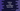# Python program to read all numbers from a file## Python program to read all numbers from a file:

In this post, we will learn how to read all numbers from a file in python and how to print all the numbers in that file. We will use one text file in this example.

### Algorithm :

Below is the algorithm we are using for this problem:

1. File name is given. Open the file in read mode.
2. Read all lines of the file.
3. Iterate through the lines one by one.
4. For each line, iterate through the characters of that line.
5. Check for each character, if it is a digit or not. If it is a digit, print the number.

### Python program:

Below is the complete python program:

``````given_file = open('input.txt', 'r')

for line in lines:
for c in line:
if c.isdigit() == True:
print('Integer found : {}'.format(c))

given_file.close()``````

### Output:

Create one file input.txt in the same folder where we have the python file containing the above code.

If the input.txt file contains the below text :

``````hello1
world 23       4
new line    5
new line one more 6

7``````

It will print:

``````Integer found : 1
Integer found : 2
Integer found : 3
Integer found : 4
Integer found : 5
Integer found : 6
Integer found : 7``````

As you can see here, it prints all the numbers it found in the file.